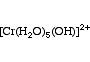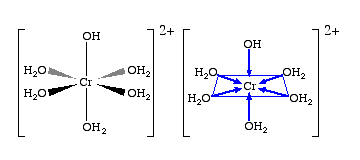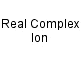Chromium(III) pentaaquahydroxy ion
[Cr(H2O)5(OH)]2+
Pentaaquahydroxychromium(III) ionCr 1 H 11 O 6 Charge = +2

Real ion.

Gram formula weight (molecular mass) = 159.07Pentaaquahydroxychromium(III) ion
is defined with respect to the entities below:
The entities below are defined with respect to:
Pentaaquahydroxychromium(III) ion
 Complex (AS/A2 generic)Real complex ion (generic)Type 23 Lewis acid/base complex (generic)VSEPR geometry = AX6 (generic)Weak acid (AS/A2 generic)chemical compound molecule metal molecular science reaction mechanism ionic material acid base geometry reactivity synthesis science knowledge chemistry Lewis